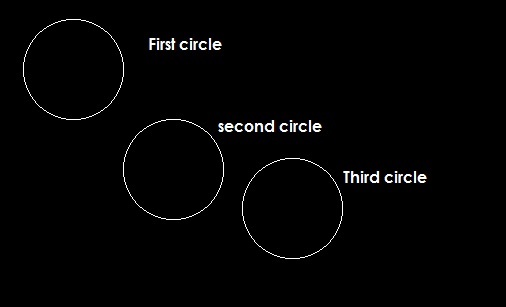# C graphics program to draw circles.

In this example we will learn how to draw circles on output screen in c programming language by using c graphics library functions?

In this example we will learn how to draw circles on output screen in c programming language by using c graphics library functions.

In this program we are using following library functions of graphics.h header file.

1. circle()
2. getmaxx()
3. getmaxy()

circle() - This library function is declared in graphics.h and used to draw a circle; it takes centre point coordinates and radius.

`circle(int x,int y, int radius);`

getmaxx() - This function returns maximum value of x coordinate.

`int getmaxx();`

getmaxy() - This function returns maximum value of y coordinate.

`int getmaxy();`

## C Code Snippet - Graphics program to draw Circles

Let’s consider the following example:

```/*C graphics program to draw circles.*/

#include <graphics.h>
#include <conio.h>

main()
{
int gd = DETECT, gm;

//init graphics
initgraph(&gd, &gm, "C:/TURBOC3/BGI");
/*
if you are using turboc2 use below line to init graphics:
initgraph(&gd, &gm, "C:/TC/BGI");
*/

//first circle from point(100,100)
circle(100,100,50);

//second circle from point(200,200)
circle(200,200,50);

//third circle from point(center,center)
circle(getmaxx()/2,getmaxy()/2,50);

getch();
closegraph();
return 0;
}
```

### Output### Explanation of functions

circle(100,100,50) – will draw a circle of 50 from point(100,100).

circle(200,200,50) – will draw a circle of 50 from point(200,200).

circle(getmaxx()/2,getmaxy()/2,50) – will draw a circle of 50 from centre of the screen.